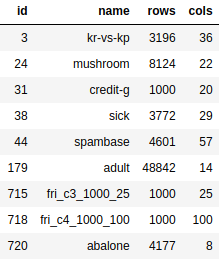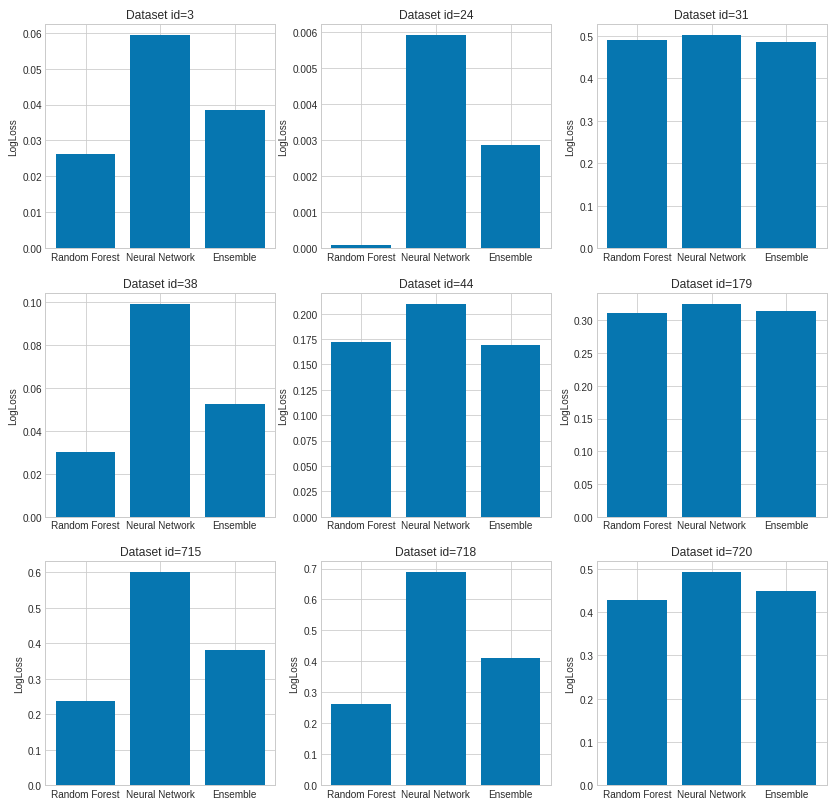#Random Forests® vs Neural Networks: Which is Better, and When?

Random Forests and Neural Network are the two widely used machine learning algorithms. What is the difference between the two approaches? When should one use Neural Network or Random Forest?

By Piotr Płoński, the founder of MLJARWhich is better: Random Forests or Neural Network? This is a common question, with a very easy answer: it depends :). I will try to show you when it is good to use Random Forests and when to use Neural Network.

First of all, Random Forests (RF) and Neural Network (NN) are different types of algorithms. The RF is the ensemble of decision trees. Each decision tree, in the ensemble, process the sample and predicts the output label (in case of classification). Decision trees in the ensemble are independent. Each can predict the final response. The Neural Network is a network of connected neurons. The neurons cannot operate without other neurons - they are connected. Usually, they are grouped in layers and process data in each layer and pass forward to next layers. The last layer of neurons is making decisions.

The Random Forests can only work with tabular data. (What is tabular data? It is data in a table format). On the other hand, Neural Network can work with many different data types:

• Tabular data
• Images (the NN become very popular after beating image classification benchmarks, for more details please read more about Convolutional Neural Networks (CNN))
• Audio data (also handled with CNN)
• Text data - can be handled by NN after preprocessing, for example with bag-of-words. In theory, RF can work with such data as well, but in real-life applications, after such preprocessing, data will become sparse and RF will be stuck.

OK, so now you have some intuition, that when you deal with images, audio or text data, you should select NN.

### What about tabular data?

In the case of tabular data, you should check both algorithms and select the better one. Simple. However, I would prefer Random Forests over Neural Network, because they are easier to use. I'll show you why.

### Random Forests vs Neural Network - data preprocessing

In theory, the Random Forests should work with missing and categorical data. However, the sklearn implementation doesn't handle this (link1link2). To prepare data for Random Forests (in python and sklearn package) you need to make sure that:

• There are no missing values in your data
• Convert categorical data into numerical

Data preprocessing for Neural Networks requires filling missing values and converting categorical data into numerical. What is more, there is a need for feature scaling. In the case of different ranges of features, there will be problems with model training. If you don't scale features into the same ranges then features with larger values will be treated as more important in the training, which is not desired. What is more, the gradients values can explode and the neurons can saturate which will make it impossible to train NN. To conclude, for Neural Network training, you need to do the following preprocessing:

• Fill missing values
• Convert categorical data into numerical
• Scale features into the same (or at least similar) range

Keep in mind, that all preprocessing that is used for preparing training data should be used in production. For NN you have more steps for preprocessing, so more steps to implement in the production system as well!

### Random Forests vs Neural Network - model training

Data is ready, we can train models.

For Random Forests, you set the number of trees in the ensemble (which is quite easy because of the more trees in RF the better) and you can use default hyperparameters and it should work.

You need some magic skills to train Neural Network well :)

• You need to define the NN architecture. How many layers to use, usually 2 or 3 layers should be enough. How many neurons to use in each layer? What activation functions to use? What weights initialization to use?
• Architecture ready. Then you need to choose a training algorithm. You can start with simple Stochastic Gradient Descent, but there are many others (RMSprop, Adagrad, Adam, Adadelta ... take a look at optimizers in Keras). Let's go with 'simple' SGD: you need to set learning rate, momentum, decay. Not enough hyperparameters? You need to set a batch size as well (batch - how many samples to show for each weights update).

You know what is funny. That each of the NN hyperparameters mentioned above can be critical. For example, you set too large learning rate or not enough neurons in second hidden-layer and your NN training will be stuck in a local minimum. Uhhh ...

### The empirical example

Stop talking, show me the results! OK, let's train models.

I will train Random Forests and Neural Network on 9 datasets from OpenML.org data repository.

The dataset description:As you see, datasets I'm using are rather small, up to a few thousand rows and several columns. I want to have small data for quick example nonetheless they cover some range of possible use cases. You can access each dataset by id from openml.org. I will use 70% of the data for training and the rest for testing with a random split.

For Random Forests and Neural Network training, I have used my open source AutoML package mljar-supervised. I prefer to use AutoML approach here because it does data preprocessing for me and tune hyperparameters.

```result = {}

for dataset_id in [3, 24, 31, 38, 44, 179, 715, 718, 720]:

result[dataset_id] = {"logloss":{"rf":[], "nn": [], "ensemble": []},
"f1":{"rf":[], "nn": [], "ensemble": []}}

x_cols = [c for c in df.columns if c != 'target']
X = df[x_cols]
y = df['target']
for repeat in range(5):
seed = 1707+repeat
X_train, X_test, y_train, y_test = \
sklearn.model_selection.train_test_split(X, y, test_size = 0.3, random_state=seed)

automl = AutoML(
total_time_limit=10*60, # 10 minutes
algorithms=["RF"],
train_ensemble=False,
verbose=False,
)
automl.fit(X_train, y_train)
y_predicted_rf = automl.predict(X_test)
result[dataset_id]["logloss"]["rf"] += [log_loss(y_test, y_predicted_rf['p_1'])]
result[dataset_id]["f1"]["rf"] += [f1_score(y_test, y_predicted_rf['label'])]

automl_nn = AutoML(
total_time_limit=10*60, # 10 minutes
algorithms=["NN"],
train_ensemble=False,
verbose=False,
)
automl_nn.fit(X_train, y_train)
y_predicted_nn = automl_nn.predict(X_test)
result[dataset_id]["logloss"]["nn"] += [log_loss(y_test, y_predicted_nn['p_1'])]
result[dataset_id]["f1"]["nn"] += [f1_score(y_test, y_predicted_nn['label'])]

y_predicted_ens = (y_predicted_rf + y_predicted_nn) / 2.0
result[dataset_id]["logloss"]["ensemble"] += [log_loss(y_test, y_predicted_ens['p_1'])]
```

In the end, I am computing simple ensemble as the average of Random Forests and Neural Network predictions.

It is worth to mention about details of training NN with AutoML. Below are hyperparameters of NN that are selected in AutoML:

```NeuralNetworkLearnerBinaryClassificationParams = {
"dense_layers": [1, 2, 3],
"dense_1_size": [4, 8, 16, 32, 64, 128],
"dense_2_size": [4, 8, 16, 32, 64],
"dense_3_size": [4, 8, 16, 32],
"dropout": [0, 0.25, 0.5, 0.75],
"learning_rate": [0.005, 0.01, 0.05, 0.1, 0.2],
"momentum": [0.85, 0.9, 0.95],
"decay": [0.0001, 0.001, 0.01],
}
```

The AutoML is creating Neural Networks with 1, 2 or 3 layers and drawing the number of neurons. For training, the SGD is used with a batch size equal to 256.

The empirical results of comparison with 5-times repetition (the lower logloss the better):Are you surprised? Maybe it is possible to get better results by Neural Network but it will require a lot of manual tuning by the expert.

The next surprising thing is the results of simple ensemble average which slightly improves the final prediction only in 3 cases (datasets with id 31, 44, 179).

### Conclusion

When you have a dilemma on which one to use, Random Forests or Neural Networks. You should decide based on the data type that you have. You should use the Neural Network for:

• Images
• Audio
• Text

If you are going to work with tabular data, it is worth to check the Random Forests first because it is easier. The Random Forests requires less preprocessing and the training process is simpler. Therefore, it is simpler to use RF in the production system. If you are not satisfied with the model performance you should try to tune and train Neural Network. There are many hyperparameters which can be tuned in NN and if you have enough knowledge and experience you can obtain very good results with NN.

Bio: Piotr Płoński is the founder of MLJAR.

Original. Reposted with permission.

RANDOM FORESTS and RANDOMFORESTS are registered marks of Minitab, LLC.

Related:

By subscribing you accept KDnuggets Privacy Policy

By subscribing you accept KDnuggets Privacy Policy

By subscribing you accept KDnuggets Privacy Policy